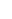# Calculate ∆ G ° for H 2 O ( g ) + 1 2 O 2 ( g ) ⇌ H 2 O 2 ( g ) at 600. K, using the following data: H 2 ( g ) + O 2 ( g ) ⇌ H 2 O 2 ( g ) K = 2.3 × 10 6 at 600 . K 2H 2 ( g ) + O 2 ( g ) ⇌ 2 H 2 O ( g ) K = 1.8 × 10 37 at 600 . K### Chemistry: An Atoms First Approach

2nd Edition
Steven S. Zumdahl + 1 other
Publisher: Cengage Learning
ISBN: 9781305079243

#### Solutions

Chapter
Section### Chemistry: An Atoms First Approach

2nd Edition
Steven S. Zumdahl + 1 other
Publisher: Cengage Learning
ISBN: 9781305079243
Chapter 16, Problem 73E
Textbook Problem
1 views

## Calculate ∆G° forH 2 O ( g ) + 1 2 O 2 ( g ) ⇌ H 2 O 2 ( g ) at 600. K, using the following data: H 2 ( g ) + O 2 ( g ) ⇌ H 2 O 2 ( g ) K =   2.3 ×   10 6   at   600 .   K 2H 2 ( g ) + O 2 ( g ) ⇌ 2 H 2 O ( g ) K =   1.8 ×   10 37   at   600 .   K

Interpretation Introduction

Interpretation: The reaction of formation of H2O2 and H2O , the value of K and T for these reactions is given. The value of ΔG° is to be calculated for the given reaction.

Concept introduction: Equilibrium constant, K , is defined as the ratio of the concentration of products to that of the reactants at equilibrium. If a given reaction is at equilibrium, the free energy change is,

ΔG=0Q=K

ΔG=ΔG°+RTln(K)

### Explanation of Solution

Given

The stated first reaction at 600K is,

H2(g)+O2(g)H2O2(g) (1)

The equilibrium constant for this reaction is,

K=2.3×106

The stated second reaction at 600K is,

2H2(g)+O2(g)2H2O(g) (2)

The equilibrium constant for this reaction is,

K=1.8×1037

Divide equation (2) on both sides by a factor 2 to get third equation.

12(2H2(g)+O2(g))H2O(g) (3)

The reaction for which ΔG° is to be calculated is,

H2O(g)+12O2(g)H2O2(g) (4)

Formula

ΔG1°=RTln(K)

Where,

• ΔG1° is the standard Gibbs free energy change for the first reaction.
• R is the gas constant (8.3145JK1mol1) .
• T is the absolute temperature.
• K is the equilibrium constant.

Substitute the values of R,T and K for first reaction in the above expression.

ΔG1°=RTln(K)=(8.3145J/K)(600K)ln(2.3×106)=73076.57J/mol_

ΔG2°=RTln(K)

Where,

• ΔG2° is the standard Gibbs free energy change for the second reaction.
• R is the gas constant (8.3145JK1mol1)

### Still sussing out bartleby?

Check out a sample textbook solution.

See a sample solution

#### The Solution to Your Study Problems

Bartleby provides explanations to thousands of textbook problems written by our experts, many with advanced degrees!

Get Started

Find more solutions based on key concepts
Explain Millikans oil-drop experiment.

General Chemistry - Standalone book (MindTap Course List)

Blood LDL values of people eating typical, meat-rich Western diets are generally higher than LDL values of vege...

Nutrition: Concepts and Controversies - Standalone book (MindTap Course List)

One millimeter contains how many m? a.10 b.100 c.1000 d.10,000

Chemistry for Today: General, Organic, and Biochemistry

Why does nuclear fusion require high temperatures?

Horizons: Exploring the Universe (MindTap Course List)

Which way do tropical cyclones spin in the Southern Hemisphere?

Oceanography: An Invitation To Marine Science, Loose-leaf Versin

Suppose the gravitational acceleration at the surface of a certain moon A of Jupiter is 2 m/s2. Moon B has twic...

Physics for Scientists and Engineers, Technology Update (No access codes included)